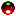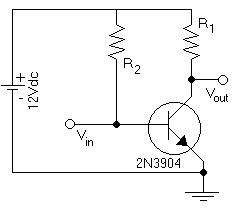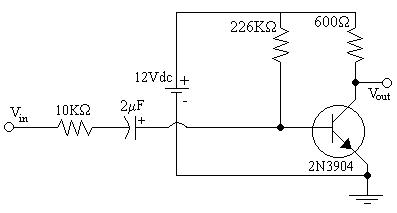EL - 250

Lab #1: The Common Emitter Amplifier

Andrew Buettner

March 6, 2003Objective

The objective of this lab is to design, and build a simple one transistor, common emitter amplifier. Then to take readings that describe it's operation. Lastly, to use those readings and compare them to theoretical values.Equipment Used

1) Trainer #1055

2) Oscilloscope #1864

3) Multimeter #25004533

5) various components from the EL - 250 Lab kitProcedure

I) Part A: Circuit Design

1) Assemble the following circuit:2) Calculate R1 and R2 using the following conditions:

a) Ic = 10mA

b) Vce = 6V

c) b = 200

II) Part B: Circuit Construction

1) Assemble the following circuit:2) Record the following:

a) Ic

b) Ib

c) Vce

d) Vb

3) Using the function generator, send a 1KHz signal to the input.

4) Connect the oscilloscope to the output and observe the result.

5) Adjust the signal so that it is at it's maximum without distortion.

6) Record the voltage at the collector, emitter, and base.Data

1) Diagram 1: Schematic 1 Enlarged view2) Table 1: R1 and R2 values

R1

600W

R2

226KW

3) Diagram 2: Schematic 2 Enlarged view4) Table 2: Resulting values from part B

Ic

Ib

Vce

Vb

6.95mA

50mA

7.82V

.73V

5) Table 3: Resulting voltages as a result of the signal

Vc

Vb

Vs

rms:

1.308V

.1901V

.2386V

Peak:

1.7V

.2V

.6VAnswers to Lab Questions

1) Q: What is the collector and base current in the amplifier, and what is the dc b?

A: Ic = 6.97mA .2% error, Ib = 49.65mA .7% error, b = 141

2) Q: What is the voltage gain between the resistor and the base?

A: 6.88x

3) Q: What is the source current using the measured voltage at the base and the source?

A: 4.85mA

4) Q: What is the input resistance to the amplifier?

A: 49.2KW

5) Q: Using the value of b experimentally obtained, determine r'e, the voltage gain, and the input resistance to the amplifier.

A: r'e = 3.66W, Av = 164, Zi = 516W

6) Q: Compare using a table, the theoretical values in Q5 to the values discovered in the earlier questions.

A:

Theoretical

Actual

Av

-164

6.88

Zi

516W

49.2KW

7) Q: What is r'e using the value of Rc and the measured gain?

A: r'e = 87.2W

8) Q: What causes the difference between the measured and theoretical values?

A: The internal resistance of the oscilloscope.Conclusions

This lab demonstrates how to build a simple, one transistor, common emitter amplifier. However, I have had difficulty explaining the vast difference between the theoretical values and the calculated ones. The experiment seemed to have been completed with a high level of accuracy; less than one percent difference between the calculated and actual current levels, however the theoretical values portray a large amount of error.Attachments

1) Original Handout

2) Original Lab Data

3) Calculations